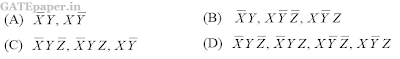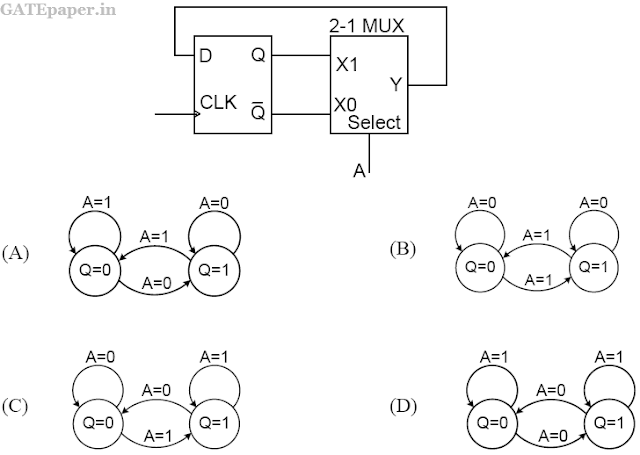### GATE 2012 ECE Video Solution on Digital Circuits (Digital Electronics)

1. Consider the given circuit. In the circuit, the race around2. The output Y of a 2-bit comparator is logic 1 whenever the 2 bit input A is greater than the 2 bit input B. The number of combinations for which the output is logic 1, is
a. 4
b. 6
c. 8
d. 10

3. In the sum of products function, f(X,Y,Z) = ∑(2,3,4,5), the prime implicants are4. The state transition diagram for the logic circuit shown is1.They produced a redesign that satisfied expectations and is user-friendly.
graphic design agency San Francisco

2.